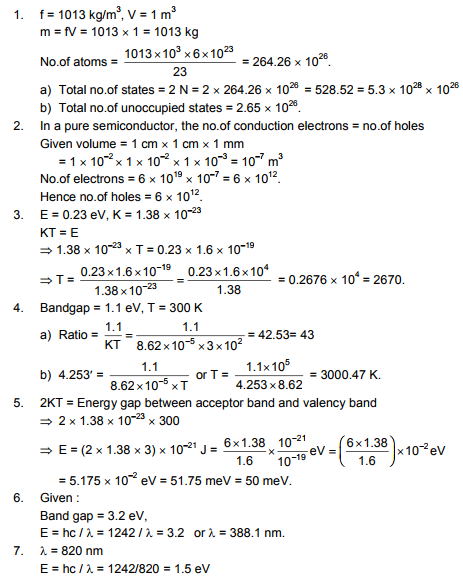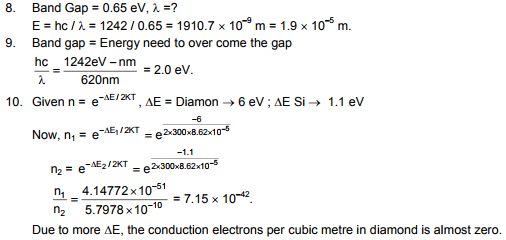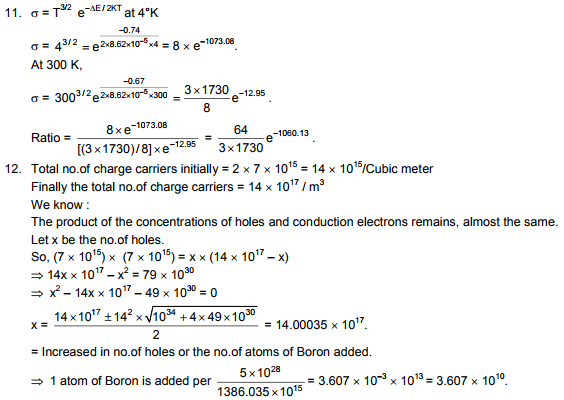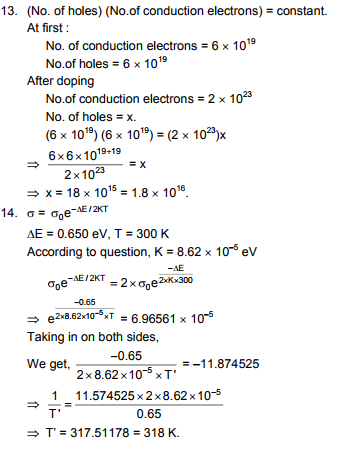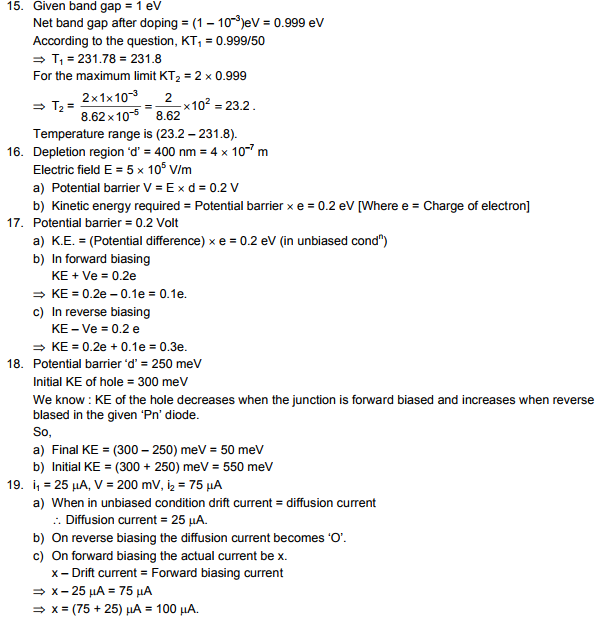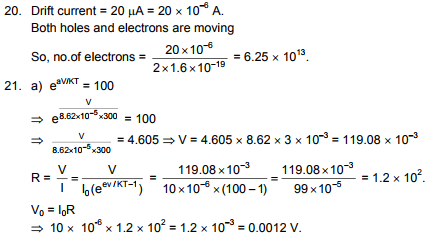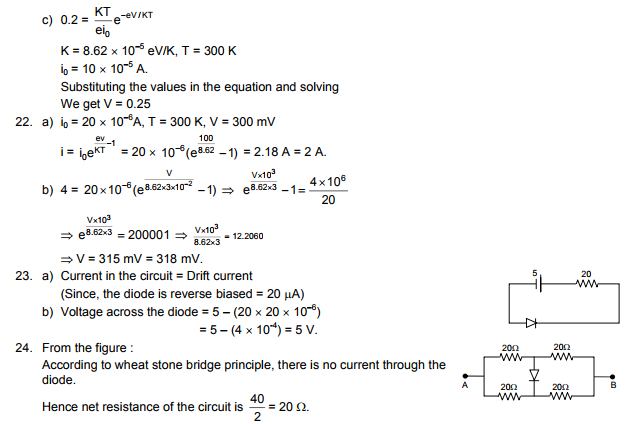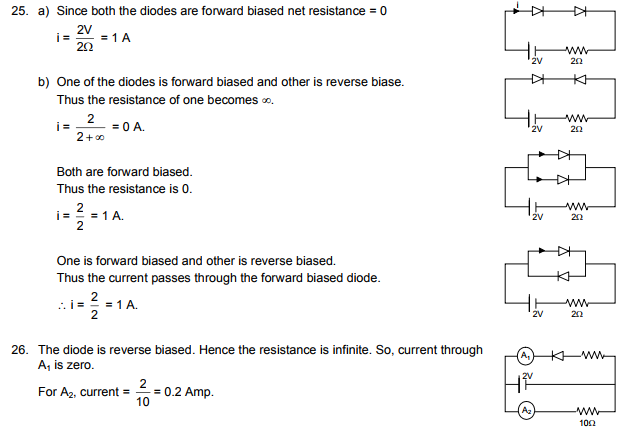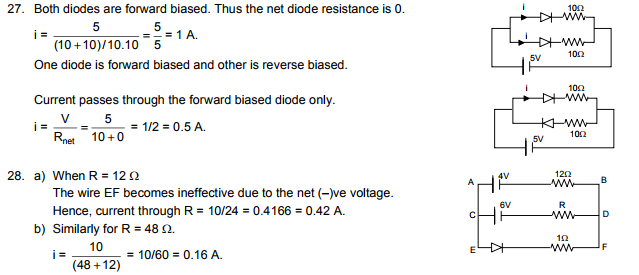# HC Verma Solutions Vol 2 Chapter 23 Semiconductor And Semiconductor Devices

HC Verma Solutions Vol 2 Chapter 23 Semiconductor and Semiconductor Devices can be used as a source of background information on topics where student’s might lack proficiency such as finding the number of conduction electrons, holes, unoccupied states in semiconductors etc. The solutions are mainly designed for class 12 students and cover the complete JEE Physics topics as well as the exercises discussed in the chapter. Some of the common questions asked in this unit are;

• There are questions related to finding the energy band between the acceptor band and valence band.
• Questions that deal with finding the energy needed to overcome the band gap.
• Students will solve questions based on unbiased, reverse and forward biasing of current and many more.

The whole idea behind preparing and offering the solutions is to help students get familiar with the question-solving pattern and integrate them into their study activities. With the solutions, students will further get an insight into important topics and understand them more clearly in the process. Students can use the solutions to also prepare well for competitive exams like JEE.

## Key Topics Related To Semiconductor In Physics

• Energy Bands in Solids
• p-type and n-type Semiconductors
• The Density of Charge Carriers and Conductivity
• p-n Junction
• p-n Junction Diode
• p-n Junction as a Rectifier
• Junction Transistors
• Logic Gates

## Important Questions In Chapter 23

1. If the conduction band of a solid is partially filled at 0 K then which category will it belong to. Will it be a semiconductor, a conductor or an insulator?

2. When we increase the temperature of the junction the drift current in a reverse-biased p-n junction also increases in magnitude. On the basis of creation of hole-electron pairs can you explain why and how this occurs?

3. In a semiconductor, you will normally find valence electrons and conduction electrons. Does a semiconductor also have ‘conduction holes’ and ‘valence holes’?

4. What condition will you find a p-type semiconductor always in?

(a) It is positively charged (b) has a negative charge (c) remains uncharged at 0 K but gets charged at high temperatures (d) no charge exists

5. When will the diffusion current in a p-n junction be greater than the drift current in magnitude?

(a) when the junction is unbiased (b) if the junction is forward-biased (c) if the junction is reverse-biased (d) such criteria do not exist

## HC Verma Solutions Vol 2 Semiconductor and Semiconductor Devices Chapter 23